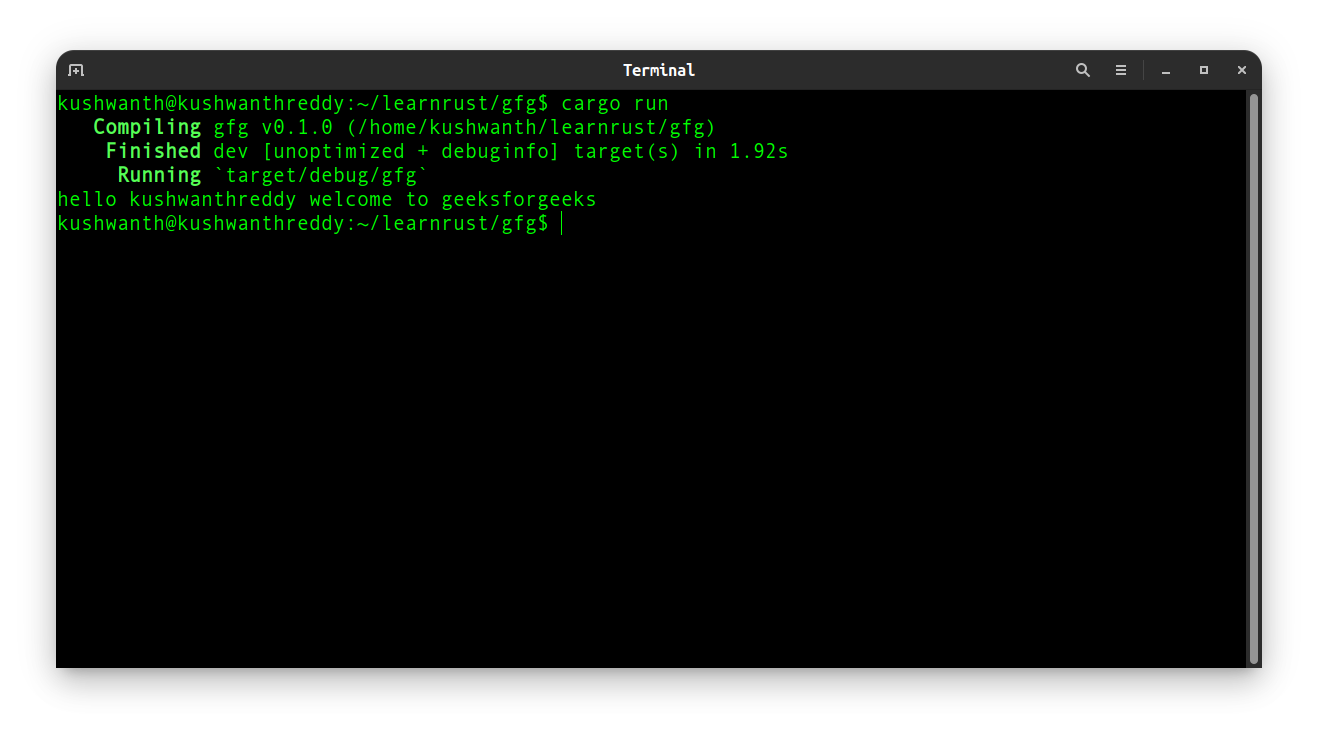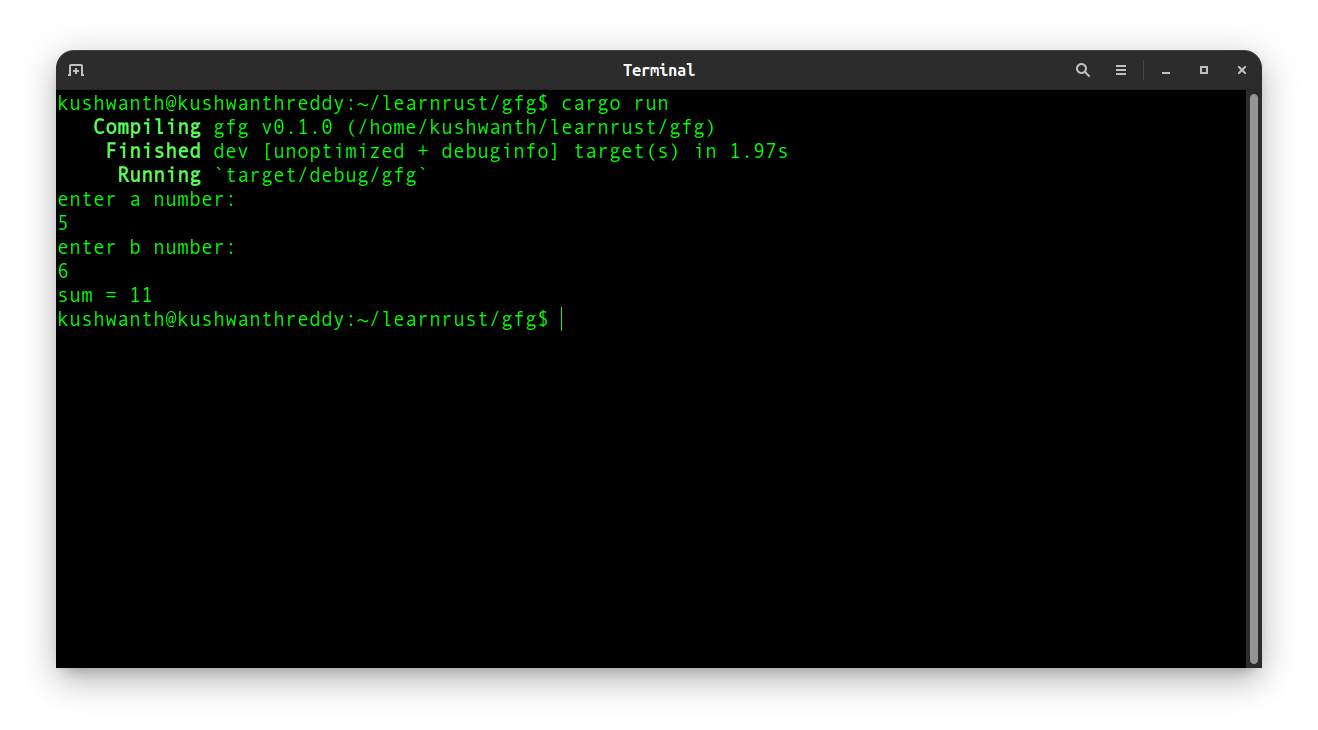Open in App
Not now

# Functions in Rust

• Last Updated : 03 Mar, 2021

Functions are the block of reusable code that can perform similar related actions. You have already seen one of the most important functions in the language: the main function, which is the entry point of many programs. You’ve also seen the “fn” keyword, which allows you to declare new functions. Rust code uses snake case as the conventional style for function and variable names. In snake case, all letters are lowercase and underscore separate words.

Syntax:

```fn functionname(arguments){
code
}```
• To create a function we need to use the fn keyword.
• The function name is written after the fn keyword
• Arguments are passed after function name inside parenthesis
• You can write function code in function block

Example:

We will look into a simple function in the below code

## Rust

 `fn` `main() {``    ``greet(``"kushwanthreddy"``);``}`` ` `fn` `greet(name: &str) {``    ``println!(``"hello {} welcome to geeksforgeeks"``,name);``}`

Output:

`hello kushwanthreddy welcome to geeksforgeeks`

In the above function declaration, we have written a greeting program that takes one argument and prints a welcome message. The parameter we have given to the greet function is a name(string datatype). We used the following approach:

• The main function has a greet function
• Greet function takes a name(string) as an argument
• Greet function prints the welcome messagefunction in rust

### Function Parameters

In the above example, we have used function without any arguments, arguments are the parameters that are special variables that are part of a function’s signature. Let us look into the below example which adds 2 numbers.

## Rust

 `use` `std::io;`` ` `fn` `main() {``    ``println!(``"enter a number:"``);``    ``let` `mut` `stra = String::new();``    ``io::stdin()``        ``.read_line(&``mut` `stra)``        ``.expect(``"failed to read input."``);``    ``println!(``"enter b number:"``);``    ``let` `mut` `strb = String::new();``    ``io::stdin()``        ``.read_line(&``mut` `strb)``        ``.expect(``"failed to read input."``);`` ` `    ``let` `a: i32 =  stra.trim().parse().expect(``"invalid input"``);``    ``let` `b: i32 =  strb.trim().parse().expect(``"invalid input"``);`` ` `    ``sum(a,b);``}`` ` `fn` `sum(x: i32, y: i32) {``    ``println!(``"sum = {}"``, x+y);``}`

Output:

```enter a number: 1
enter b number: 2

sum = 3```

As above greet program in the above program, sum function in this program also takes 2 arguments which are generally integers and output is the sum of the integers given in the argument. Here we will use the below approach:

• The program asks for input a
• The program asks for input b
• Sum program gets executed while taking a, b as arguments
• Sum program prints the sum of a, bfunction with arguments

#### Building a simple calculator in RUST

We will build a simple calculator using the function, function arguments, and conditional statements. For this we will use the below approach:

• The program asks for number a
• The program asks for number b
• The program asks to choose what to do with numbers whether their sum, difference, product, or reminder
• According to user input respective calculation is the calculator
• The sum function calculates the sum
• The sub function calculates the difference
• The mul function calculates the product
• The quo function finds out the quotient
• The rem function finds out the remainder
• For invalid argument program exits with a message “invalid”

## Rust

 `use` `std::io;``use` `std::process::``exit``;`` ` `fn` `main() {``    ``println!(``"enter a number:"``);``    ``let` `mut` `stra = String::new();``    ``io::stdin()``        ``.read_line(&``mut` `stra)``        ``.expect(``"failed to read input."``);``    ``println!(``"enter b number:"``);``    ``let` `mut` `strb = String::new();``    ``io::stdin()``        ``.read_line(&``mut` `strb)``        ``.expect(``"failed to read input."``);`` ` `    ``let` `a: i32 =  stra.trim().parse().expect(``"invalid input"``);``    ``let` `b: i32 =  strb.trim().parse().expect(``"invalid input"``);``     ` `      ``println!("choose your calculation: \n1.sum``             ``\n2.difference``             ``\n3.product``             ``\n4.quotient``             ``\n5.remainder\n");``              ` `      ``let` `mut` `choose = String::new();``    ``io::stdin()``        ``.read_line(&``mut` `choose)``        ``.expect(``"failed to read input."``);``    ``let` `c: i32 =  choose.trim().parse().expect(``"invalid input"``);``   ` `    ``// Select Operation using conditionals``      ``if` `c==1{sum(a,b);}``      ``else` `if` `c==2{sub(a,b);}``      ``else` `if` `c==3{mul(a,b);}``      ``else` `if` `c==4{quo(a,b);}``      ``else` `if` `c==5{rem(a,b);}``      ``else``{println!(``"Invalid argument"``);``exit``(1);}``}`` ` `// Sum function``fn` `sum(x: i32, y: i32) {``    ``println!(``"sum = {}"``, x+y);``}`` ` `// Difference function``fn` `sub(x: i32, y: i32) {``    ``println!(``"difference = {}"``, x-y);``}`` ` `// Product function``fn` `mul(x: i32, y: i32) {``    ``println!(``"product = {}"``, x*y);``}`` ` `// Division function``fn` `quo(x: i32, y: i32) {``    ``println!(``"quotient = {}"``, x/y);``}`` ` `// Remainder function``fn` `rem(x: i32, y: i32) {``    ``println!(``"remainder = {}"``, x%y);``}`

Output:

```enter a number:
2
enter b number:
4